Study Materials: ncert solutions

Our ncert solutions for Exercise 12.1 Class 9 maths 12. Herons Formula - ncert solutions - Toppers Study is the best material for English Medium students cbse board and other state boards students.

Solutions ⇒ Class 9th ⇒ Mathematics ⇒ 12. Herons Formula

# Exercise 12.1 Class 9 maths 12. Herons Formula - ncert solutions - Toppers Study

Topper Study classes prepares ncert solutions on practical base problems and comes out with the best result that helps the students and teachers as well as tutors and so many ecademic coaching classes that they need in practical life. Our ncert solutions for Exercise 12.1 Class 9 maths 12. Herons Formula - ncert solutions - Toppers Study is the best material for English Medium students cbse board and other state boards students.

## Exercise 12.1 Class 9 maths 12. Herons Formula - ncert solutions - Toppers Study

CBSE board students who preparing for class 9 ncert solutions maths and Mathematics solved exercise chapter 12. Herons Formula available and this helps in upcoming exams 2023-2024.

### You can Find Mathematics solution Class 9 Chapter 12. Herons Formula

• All Chapter review quick revision notes for chapter 12. Herons Formula Class 9
• NCERT Solutions And Textual questions Answers Class 9 Mathematics
• Extra NCERT Book questions Answers Class 9 Mathematics
• Importatnt key points with additional Assignment and questions bank solved.

NCERT Solutions do not only help you to cover your syllabus but also will give to textual support in exams 2023-2024 to complete exercise 12.1 maths class 9 chapter 12 in english medium. So revise and practice these all cbse study materials like class 9 maths chapter 12.1 in english ncert book. Also ensure to repractice all syllabus within time or before board exams for ncert class 9 maths ex 12.1 in english.

See all solutions for class 9 maths chapter 12 exercise 12 in english medium solved questions with answers.

### Exercise 12.1 class 9 Mathematics Chapter 12. Herons Formula

• Exercise 12.1 Class 9 Maths 12. Herons Formula - Ncert Solutions - Toppers Study
• Class 9 Ncert Solutions
• Solution Chapter 12. Herons Formula Class 9
• Solutions Class 9
• Chapter 12. Herons Formula Exercise 12.1 Class 9

## Exercise 12.1 Class 9 maths 12. Herons Formula - ncert solutions - Toppers Study

Exercise 12.1

Q1. A traffic signal board, indicating ‘SCHOOL AHEAD’, is an equilateral triangle with side ‘a’. Find the area of the signal board, using Heron’s formula. If its perimeter is 180 cm, what will be the area of the signal board?

Solution:Perimeter of traffic signal board = 180 cm

Side of traffic signal board

Using equation (1), area of traffic signal board

Q2:The triangular side walls of a flyover have been used for advertisements.The sides of the walls are 122 m, 22 m and 120 m (see Fig. 12.9). The advertisements yield an earning of Rs 5000 per m2 per year. A company hired one of its walls for 3 months. How much rent did it pay?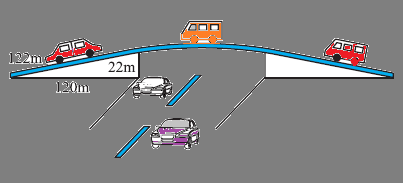Solution:

The sides of the triangle a,b,c are of 122 m, 22 m, and 120 m respectively.

Perimeter of triangle = (122 + 22 + 120) m

2s = 264 m

s = 132 m

By Heron's formula,

Rent of 1 m2 area per year = Rs 5000

Rent of 1 m2 area per month = Rs

Rent of 1320 m2 area for 3 months =

= Rs (5000 × 330) = Rs 1650000

Therefore, the company had to pay Rs 1650000

Q3: There is a slide in the park. One of its side walls has been painted in the same color with a message “KEEP THE PARK GREEN AND CLEAN” (see the given figure). If the sides of the wall are 15m, 11m, and 6m, find the area painted in color.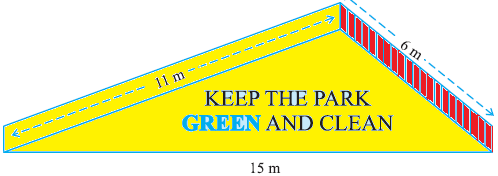It can be observed that the area to be painted in colour is a triangle, having its sides as 11 m, 6 m, and 15 m.

Perimeter of such a triangle = (11 + 6 + 15) m

s = 32 m

s = 16 m

By Heron's formula

Area of triangle=Therefore, the area painted in color is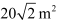Q4 :  Find the area of a triangle two sides of which are 18 cm and 10 cm              and the perimeter is 42 cm.

Let the third side of the triangle be x.

Perimeter of the given triangle = 42 cm

18 cm + 10 cm + c = 42

c = 14 cm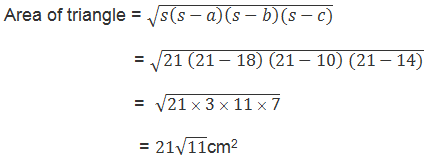5. Sides of a triangle are in the ratio of 12: 17: 25 and its perimeter is 540cm. Find its area of triangle.

Solution:

: Let the common ratio between the sides of the given triangle be x.

Therefore, the side of the triangle will be 12x, 17x, and 25x.

Perimeter of this triangle = 540 cm

12x + 17x + 25x = 540 cm

54x = 540 cm

x = 10 cm

Sides of the triangle will be 120 cm, 170 cm, and 250 cm.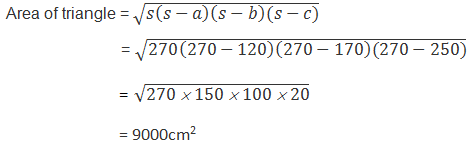Q6 :  An isosceles triangle has perimeter 30 cm and each of the equal                sides is 12 cm. Find the area of the triangle.

Solution:

Let the third side of this triangle be x.

Perimeter of triangle = 30 cm

12 cm + 12 cm + x = 30 cm

x = 6 cm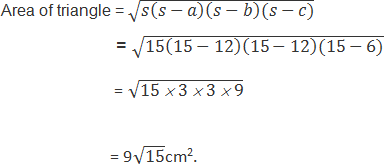##### Other Pages of this Chapter: 12. Herons Formula

Important Study materials for classes 06, 07, 08,09,10, 11 and 12. Like CBSE Notes, Notes for Science, Notes for maths, Notes for Social Science, Notes for Accountancy, Notes for Economics, Notes for political Science, Noes for History, Notes For Bussiness Study, Physical Educations, Sample Papers, Test Papers, Mock Test Papers, Support Materials and Books.Mathematics Class - 11th

NCERT Maths book for CBSE Students.

books

## Study Materials List:

##### Solutions ⇒ Class 9th ⇒ Mathematics
1. Number Systems
2. Polynomials
3. Coordinate Geometry
4. Linear Equation In Two Variables
5. Introduction To Euclid’s Geometry
6. Lines and Angles
7. Triangles